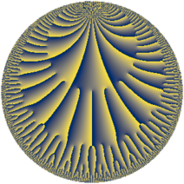# Properties

 Label 21.30.cLevel $21$ Weight $30$ Character orbit 21.c Rep. character $\chi_{21}(20,\cdot)$ Character field $\Q$ Dimension $76$ Newform subspaces $1$ Sturm bound $80$ Trace bound $0$

# Related objects

## Defining parameters

 Level: $$N$$ $$=$$ $$21 = 3 \cdot 7$$ Weight: $$k$$ $$=$$ $$30$$ Character orbit: $$[\chi]$$ $$=$$ 21.c (of order $$2$$ and degree $$1$$) Character conductor: $$\operatorname{cond}(\chi)$$ $$=$$ $$21$$ Character field: $$\Q$$ Newform subspaces: $$1$$ Sturm bound: $$80$$ Trace bound: $$0$$

## Dimensions

The following table gives the dimensions of various subspaces of $$M_{30}(21, [\chi])$$.

Total New Old
Modular forms 80 80 0
Cusp forms 76 76 0
Eisenstein series 4 4 0

## Trace form

 $$76 q - 20401094660 q^{4} + 1962751543872 q^{7} - 89581451225964 q^{9} + O(q^{10})$$ $$76 q - 20401094660 q^{4} + 1962751543872 q^{7} - 89581451225964 q^{9} - 116668222909200048 q^{15} + 4964525139713333892 q^{16} + 1542346202347854996 q^{18} + 33840646982361357348 q^{21} - 9870996616978150672 q^{22} + 3225406746743103424612 q^{25} - 3181907581338093199564 q^{28} + 5113912044496410095244 q^{30} - 85758641407134848258352 q^{36} - 17812771227715200149976 q^{37} + 388095283792424641491696 q^{39} + 804036587819434104664956 q^{42} + 2391536152977907871782320 q^{43} - 1070985628070611677307000 q^{46} - 512656982878691867441540 q^{49} - 961431915936904000664400 q^{51} + 20345422731681279280765248 q^{57} - 40096240109469024574169272 q^{58} - 164991837955115951799442596 q^{60} - 360764839802574990409097184 q^{63} - 1608343949909674725081473028 q^{64} + 483978610969894046870693264 q^{67} - 656026306765550013508448520 q^{70} + 1937172856654554597960695124 q^{72} - 8054141680325499868559088636 q^{78} - 11740735193468750125742145952 q^{79} + 18477545939654446572971367132 q^{81} - 7249045143271847568431965452 q^{84} + 14576359553686844022629140848 q^{85} - 1685535263940707754634995584 q^{88} + 44135166284535829618726469040 q^{91} + 226499776928895503792863540632 q^{93} - 231093384978708762520959066624 q^{99} + O(q^{100})$$

## Decomposition of $$S_{30}^{\mathrm{new}}(21, [\chi])$$ into newform subspaces

Label Dim. $$A$$ Field CM Traces $q$-expansion
$$a_2$$ $$a_3$$ $$a_5$$ $$a_7$$
21.30.c.a $$76$$ $$111.884$$ None $$0$$ $$0$$ $$0$$ $$19\!\cdots\!72$$